# Angle Problems

#### Number of problems found: 626

• Roof 8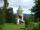How many liters of air are under the roof of tower which has the shape of a regular six-sided pyramid with a 3,6-meter-long bottom edge and a 2,5-meter height? Calculate the supporting columns occupy about 7% of the volume under the roof.
• The towerThe observer sees the tower's base 96 meters high at a depth of 30 degrees and 10 minutes and the top of the tower at a depth of 20 degrees and 50 minutes. How high is the observer above the horizontal plane on which the tower stands?
• Center of gravityIn the isosceles triangle ABC is the ratio of the lengths of AB and the height to AB 10:12. The arm has a length of 26 cm. If the center of gravity T of triangle ABC find area of triangle ABT.
• Triangle in a squareIn a square ABCD with side a = 6 cm, point E is the center of side AB and point F is the center of side BC. Calculate the size of all angles of the triangle DEF and the lengths of its sides.
• Minute handWhat is the distance the minute hand of the clock travels in 12 minutes, if the diameter of the clock is 30 cm and the hand extends to a distance of 2 cm from the edge of the clock?
• Office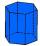Office building was built in the shape of a regular hexagon inscribed in a circle with a radius of 12 m. The height of the walls is 7m. How much CZK cost plastering the walls of the building, if per 1 m square cost CZK 400?
• Distance of pointsA regular quadrilateral pyramid ABCDV is given, in which edge AB = a = 4 cm and height v = 8 cm. Let S be the center of the CV. Find the distance of points A and S.
• GarageThere are two laths in the garage opposite one another: one 2 meters long and the second 3 meters long. They fall against each other and stay against the opposite walls of the garage and both laths cross 70 cm above the garage floor. How wide is the garag
• Tree shadowTree perpendicular to the horizontal surface has a shadow 8.32 meters long. At the same time meter rod perpendicular to the horizontal surface has shadow 64 cm long. How tall is tree?
• Hexagonal pyramidCalculate the surface area of a regular hexagonal pyramid with a base inscribed in a circle with a radius of 8 cm and a height of 20 cm.
• Latitude and longitude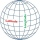A helicopter is flying from Hong Kong to Jakarta. The latitude of Jakarta is -6.20, and its longitude is 106.80. The latitude of Hong Kong is 22.27, and its longitude is 114.15. What is the helicopters net change in latitude and longitude as it travels fr
• Circle sectionEquilateral triangle with side 33 is inscribed circle section whose center is in one of the vertices of the triangle and the arc touches the opposite side. Calculate: a) the length of the arc b) the ratio betewwn the circumference to the circle sector and
• Hexagonal pyramidRegular hexagonal pyramid has dimensions: length edge of the base a = 1.8 dm and the height of the pyramid = 2.4 dm. Calculate the surface area and volume of a pyramid.
• Eq triangle minus arcsIn an equilateral triangle with a 2cm side, the arcs of three circles are drawn from the centers at the vertices and radii 1cm. Calculate the content of the shaded part - a formation that makes up the difference between the triangle area and circular cuts
• Constant Angular Acceleration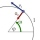The particle began to move from rest along a circle with a constant angular acceleration. After five cycles (n = 5), its angular velocity reached the value ω = 12 rad/s. Calculate the magnitude of the angular acceleration ε of this motion and the time int
• Trapezoid - diagonalA trapezoid has a length of diagonal AC crossed with diagonal BD in the ratio 2:1. The triangle created by points A, cross point of diagonals S and point D has area 164 cm2. What is the area of the trapezoid?
• Angles and sides of the triangle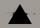Triangle ABC has a circumference of 26 cm. Lengths of the sides are as follows: a = 11.2 cm; b = 6.5 cm. Arrange the interior angles in order of their size. ?
• Inscribed circleXYZ is right triangle with right angle at the vertex X that has inscribed circle with a radius 5 cm. Determine area of the triangle XYZ if XZ = 14 cm.
• Hexagonal prismThe base of the prism is a regular hexagon consisting of six triangles with side a = 12 cm and height va = 10.4 cm. The prism height is 5 cm. Find the volume and surface of the prism.
• Octagonal tank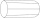The tank has the shape of a regular octagonal prism without an upper base. The base edge has a = 3m, the side edge b = 6m. How much metal sheet is needed to build the tank? Do not think about losses or sheet thickness.

Do you have an interesting mathematical word problem that you can't solve it? Submit a math problem, and we can try to solve it.

We will send a solution to your e-mail address. Solved examples are also published here. Please enter the e-mail correctly and check whether you don't have a full mailbox.

Please do not submit problems from current active competitions such as Mathematical Olympiad, correspondence seminars etc...